# The TOP - 29 term deposits for 3 years12.02.2020

## Bankchart.com.au has surveyed the terms of term deposits for 3 years in the national currency. The survey covered all the deposits with any form of interests repayment, regardless of the replenishment option or the deposit partial withdrawal option. Our website's analysts have studied the loan offers from all Australian banks. Review date - 12.02.2020

As of 25 January 2020 the deposits under review have been offered by 29 banks.  The average interest rate under such deposits equals 1,25 % p.a. The total number of the deposits programs available for the specified terms is 110.

The table below shows the best offers by each bank selected by Bankchart.com.au (in terms of profitability) for the aforementioned terms.

### The TOP-29 term deposits for 3 years, as of 25 January 2020

#

Name

Interest Rate, %

1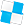Police Bank

«Term Deposits»

1,60

interest rate, %

rate type

fixed

interest periodicity

every 6 months

minimum sum

\$ 10 000,00

2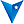BankVic

«50+ investment account»

1,60

interest rate, %

rate type

fixed

interest periodicity

annually

minimum sum

\$ 50 000,00

3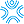Bank First

«Term Deposits»

1,60

interest rate, %

rate type

fixed

interest periodicity

monthly

minimum sum

\$ 50 000,00

4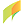G&C Mutual Bank

«Term Deposits»

1,55

interest rate, %

rate type

fixed

interest periodicity

at maturity, annually

minimum sum

\$ 1 000,00

5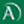Arab Bank

«Fixed Term Deposit»

1,50

interest rate, %

rate type

fixed

interest periodicity

at maturity

minimum sum

\$ 50 000,00

6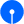State Bank of India

«Term Deposits»

1,50

interest rate, %

rate type

fixed

interest periodicity

at maturity

minimum sum

\$ 100,00

7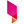Bank Australia

«Term Deposits»

1,50

interest rate, %

rate type

fixed

interest periodicity

at maturity

minimum sum

\$ 500,00

8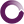Beyond Bank Australia

«Term Deposits»

1,50

interest rate, %

rate type

fixed

interest periodicity

annually

minimum sum

\$ 200 000,00

9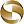Mega International Commercial Bank

«Term Deposits»

1,50

interest rate, %

rate type

fixed

interest periodicity

annually

minimum sum

\$ 1 000,00

10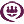RACQ

«Term Deposit»

1,50

interest rate, %

rate type

fixed

interest periodicity

quarterly

minimum sum

\$ 500,00

11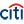Citibank

«Term deposit»

1,46

interest rate, %

rate type

fixed

interest periodicity

monthly

minimum sum

\$ 10 000,00

12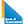Bank of Queensland

«Term deposit»

1,45

interest rate, %

rate type

fixed

interest periodicity

at maturity

minimum sum

\$ 5 000,00

13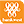Bank of Western Australia

«Online Term Deposit»

1,45

interest rate, %

rate type

fixed

interest periodicity

at maturity, annually

minimum sum

\$ 5 000,00

14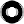Macquarie Bank

«Term Deposit»

1,45

interest rate, %

rate type

fixed

interest periodicity

monthly

minimum sum

\$ 0,00

15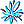AMP Bank

«Term Deposits»

1,40

interest rate, %

rate type

fixed

interest periodicity

at maturity

minimum sum

\$ 500 000,00

16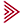Bendigo and Adelaide Bank

«Term deposit»

1,40

interest rate, %

rate type

fixed

interest periodicity

annually

minimum sum

\$ 5 000,00

17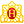Heritage Bank

«Term Deposits»

1,40

interest rate, %

rate type

fixed

interest periodicity

annually

minimum sum

\$ 1 000,00

18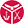Defence Bank

«Defence Bank Super Term Deposits»

1,40

interest rate, %

rate type

fixed

interest periodicity

at maturity

minimum sum

\$ 10 000,00

19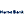Hume Bank

«Term Deposits»

1,35

interest rate, %

rate type

fixed

interest periodicity

at maturity, annually

minimum sum

\$ 5 000,00

20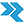Rural Bank

«Long Term Deposit»

1,35

interest rate, %

rate type

fixed

interest periodicity

annually

minimum sum

\$ 150 000,00

21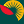Suncorp Bank

«Term Deposit»

1,35

interest rate, %

rate type

fixed

interest periodicity

at maturity

minimum sum

\$ 1 000 000,00

22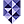Bank of Melbourne

«Term Deposits»

1,30

interest rate, %

rate type

fixed

interest periodicity

annually

minimum sum

\$ 1 000,00

23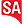Bank SA

«Term Deposits»

1,30

interest rate, %

rate type

fixed

interest periodicity

annually

minimum sum

\$ 1 000,00

24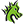St. George Bank

«Term Deposits»

1,30

interest rate, %

rate type

fixed

interest periodicity

annually

minimum sum

\$ 1 000,00

25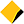Commonwealth Bank of Australia

«Term Deposits»

1,25

interest rate, %

rate type

fixed

interest periodicity

annually

minimum sum

\$ 50 000,00

26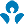Australia and New Zealand (ANZ)

1,20

interest rate, %

rate type

fixed

interest periodicity

every 6 months

minimum sum

\$ 5 000,00

27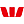Westpac Bank

«Term Deposit»

1,20

interest rate, %

rate type

fixed

interest periodicity

monthly

minimum sum

\$ 5 000,00

28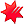National Australia Bank

«NAB Term Deposits»

1,15

interest rate, %

rate type

fixed

interest periodicity

at maturity, annually

minimum sum

\$ 5 000,00

29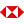HSBC Bank

«Term Deposits»

1,10

interest rate, %

rate type

fixed

interest periodicity

at maturity

minimum sum

\$ 5 000,00

To view the up-to-date rating of the deposits at our website here.